MathScore EduFighter is one of the best math games on the Internet today. You can start playing for free!

## Percentage Pictures - Sample Math Practice Problems

The math problems below can be generated by MathScore.com, a math practice program for schools and individual families. References to complexity and mode refer to the overall difficulty of the problems as they appear in the main program. In the main program, all problems are automatically graded and the difficulty adapts dynamically based on performance. Answers to these sample questions appear at the bottom of the page. This page does not grade your responses.

See some of our other supported math practice problems.

### Complexity=3, Mode=100grid

Determine what percentage of the picture is shaded. If necessary, round to the nearest percentage.

 1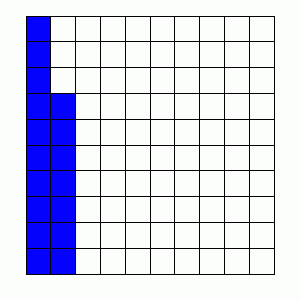% 2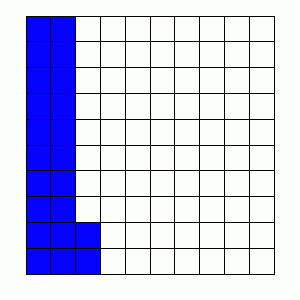%

### Complexity=3, Mode=10square

Determine what percentage of the picture is shaded. If necessary, round to the nearest percentage.

 1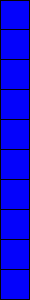% 2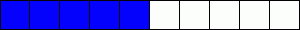%

### Complexity=5, Mode=polygon

Determine what percentage of the picture is shaded. If necessary, round to the nearest percentage.

 1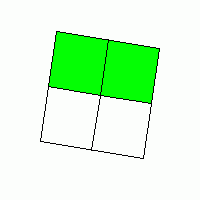% 2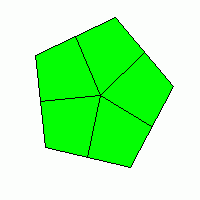%

### Complexity=9, Mode=polygon

Determine what percentage of the picture is shaded. If necessary, round to the nearest percentage.

 1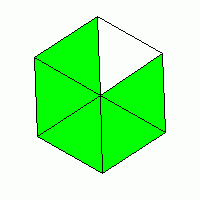% 2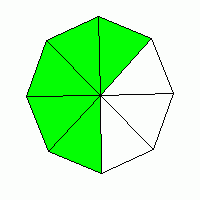%

### Complexity=15, Mode=polygon

Determine what percentage of the picture is shaded. If necessary, round to the nearest percentage.

 1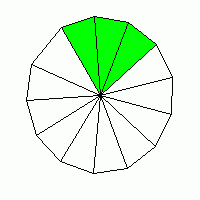% 2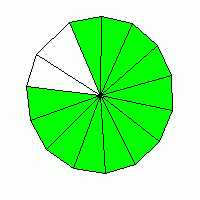%

### Complexity=3, Mode=100grid

Determine what percentage of the picture is shaded. If necessary, round to the nearest percentage.

1%
Solution
17 boxes out of 100 are shaded.
Percentage is a ratio out of 100, so the answer is 17%
2%
Solution
22 boxes out of 100 are shaded.
Percentage is a ratio out of 100, so the answer is 22%

### Complexity=3, Mode=10square

Determine what percentage of the picture is shaded. If necessary, round to the nearest percentage.

1%

Solution
2%

Solution
5 out of 10 boxes are shaded.
5/10 = 0.5
Multiply the decimal by 100 to get a percentage.
0.5 × 100 = 50%

Alternate Solution
5 out of 10 boxes are shaded.
Multiply by 10 to make the ratio out of 100: 50/100
Percentage is a ratio out of 100, so the answer is 50%

### Complexity=5, Mode=polygon

Determine what percentage of the picture is shaded. If necessary, round to the nearest percentage.

1%
Solution
2 out of 4 parts are shaded.
2/4 = 0.5
Multiply the decimal by 100 to get a percentage.
0.5 × 100 = 50%

Alternate Solution
This fraction simplifies to 1/2
Half of something is 50%

2%
Solution

### Complexity=9, Mode=polygon

Determine what percentage of the picture is shaded. If necessary, round to the nearest percentage.

1%
Solution
5 out of 6 parts are shaded.
5/6 = 0.83333333333333
Multiply the decimal by 100 to get a percentage.
0.83333333333333 × 100 = 83.333333333333%
Round 83.333333333333 to 83%
2%
Solution
5 out of 8 parts are shaded.
5/8 = 0.625
Multiply the decimal by 100 to get a percentage.
0.625 × 100 = 62.5%
Round 62.5 to 63%

### Complexity=15, Mode=polygon

Determine what percentage of the picture is shaded. If necessary, round to the nearest percentage.

1%
Solution
3 out of 13 parts are shaded.
3/13 = 0.23076923076923
Multiply the decimal by 100 to get a percentage.
0.23076923076923 × 100 = 23.076923076923%
Round 23.076923076923 to 23%
2%
Solution
12 out of 14 parts are shaded.
12/14 = 0.85714285714286
Multiply the decimal by 100 to get a percentage.
0.85714285714286 × 100 = 85.714285714286%
Round 85.714285714286 to 86%

Alternate Solution
This fraction simplifies to 6/7
6/7 = 0.85714285714286
Multiply the decimal by 100 to get a percentage.
0.85714285714286 × 100 = 85.714285714286%
Round 85.714285714286 to 86%